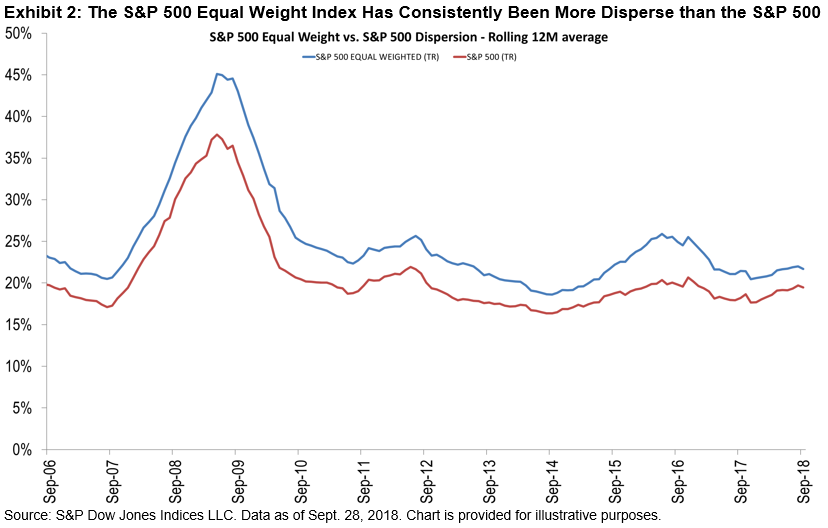# Surprising but Explainable

Equal-weight indices have a small-cap tilt. Therefore, one might naturally assume that the volatility of equal-weight indices is higher than that of their cap-weighted counterparts. Surprisingly, this is not always the case, and we can understand why using the lens of dispersion and correlation.

Exhibit 1 shows that the volatility of the S&P 500® Equal Weight Index was about 1% less than that of the (cap-weighted) S&P 500, as of the end of Q3 2018. (A key driver of this difference was the Information Technology sector, as the S&P 500 Equal Weight Information Technology Index was 1.6% less volatile than the S&P 500 Information Technology. Readers who are particularly interested in comparing equal-weight and cap-weighted sectors would enjoy perusing our new U.S. Equal Weight Sectors Dashboard.)Exhibit 2 shows that the dispersion of the S&P 500 Equal Weight Index was consistently higher than that of the S&P 500. This is not a surprising outcome since, as a function of equal weighting, the index has higher weights in smaller, more idiosyncratic stocks.Exhibit 3 provides two important insights about the relative correlation of the S&P 500 Equal Weight Index and its cap-weighted parent:

• The weighted average correlation of the equal weight index is consistently lower than that of the S&P 500.
• The spread between the correlation of the two indices has recently widened.Other things equal, volatility rises as either dispersion or correlation rise. (This interaction helps us, for example, to understand the volatility of the new Communication Services sector.) The equal weight index’s higher dispersion drives volatility higher, but is balanced by its (unusually) lower correlations. This combination caused the S&P 500 Equal Weight Index’s volatility to drop below that of the S&P 500, an unexpected but justifiable outcome.